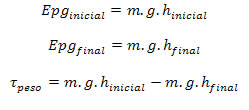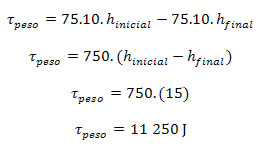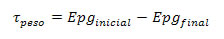Mechanics

# Work of the weight force

We know that work is the energy expended to perform a task. Based on this, it is possible to define the change in gravitational potential energy as the work of the weight force.

In Physics, we saw that the work of any force is equivalent to the measure of the amount of energy transformed or transferred from one body (system) to another, or to the amount of energy transformed within the same system. Based on this definition, we will determine the value of work in a situation that we will describe next.

A person with a mass of 75 kg is in free fall during bungee jumping and is therefore falling. During the first 15 m of the fall, the falling speed of the person is equal to 16 m/s, that is, 57.6 km/h. Consider the acceleration due to gravity 10 m/s 2 .

For this bungee jump , we will calculate the work value of the weight force through the initial potential energy and final potential energy. So we have:In the above equations, we have:

E pg – gravitational potential energy;

m – mass;

g – acceleration due to gravity;

Don’t stop now… There’s more after the publicity 😉

h – height;

τ – work of the weight force.

Substituting the values ​​we know, we have:The positive sign of work means that the athlete gains kinetic energy with the work of the weight force, as the distance between him and the Earth decreases, that is, his gravitational potential energy decreases.

The unit of measure of work in the International System of Units SI ) is the joule (J), since it can be calculated from the difference in energies. When any object is subject only to the action of gravitational force, the work of this force is the measure of the transformation of kinetic potential energy.

Since the change in kinetic energy of a falling object corresponds to the decrease in its gravitational potential energy, we can calculate work using the difference between the initial potential energy and the final potential energy.